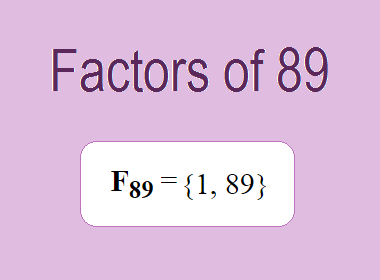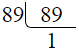# Factors of 89The factors of 89 are 1 and 89 i.e. F89 = {1, 89}. The factors of 89 are all the numbers that can divide 89 without leaving a remainder. 89 is a prime number, so it is divisible by 1 and 89 only.

We can check if these numbers are factors of 89 by dividing 89 by each of them. If the result is a whole number, then the number is a factor of 89. Let's do this for each of the numbers listed above:

·        1 is a factor of 89 because 89 divided by 1 is 89.

·        89 is a factor of 89 because 89 divided by 89 is 1.

## Properties of the Factors of 89

The factors of 89 have some interesting properties. One of the properties is that the sum of the factors of 89 is equal to 90. We can see this by adding all the factors of 89 together:

1 + 89 = 90

Another property of the factors of 89 is that the only prime factor of 89 is 89 itself.

********************

********************

## Applications of the Factors of 89

The factors of 89 have several applications in mathematics. One of the applications is in finding the highest common factor (HCF) of two or more numbers. The HCF is the largest factor that two or more numbers have in common. For example, to find the HCF of 89 and 178, we need to find the factors of both numbers and identify the largest factor they have in common. The factors of 89 are 1, and 89. The factors of 178 are 1, 2, 89, and 178. The largest factor that they have in common is 89. Therefore, the HCF of 89 and 178 is 89.

Another application of the factors of 89 is in prime factorization. Prime factorization is the process of expressing a number as the product of its prime factors. The prime factor of 89 is 89 since it is only the prime number that can divide 89 without leaving a remainder. Therefore, we can express 89 as:

89 = 89

We can do prime factorization by division method as given below,89 = 89

Since 89 is a prime number, there is no factor tree of 89.

## Conclusion

The factors of 89 are the numbers that can divide 89 without leaving a remainder. The factors of 89 are 1, and 89. The factors of 89 have some interesting properties, such as having a sum of 90. The factors of 89 have several applications in mathematics, such as finding the highest common factor and prime factorization.